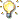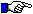# Sampling and signal reconstruction

### Procedure for Sampling experiment

Exp-4(a) Sampling of band limited signal : Nyquist theorem

Exp-4(b) Sampling of non bandlimited signal : Anti aliasing filter

Exp-4(c) Signal reconstruction from its samples

Exp-4(d) Frequency domain sampling : DFT

Exp-4(e) Spectral analysis using DFT•       Run the experiment by pressing "" button.
•

• In this experiment we verify the Nyquist sampling theorem.

• Select a continuous-time signal x(t) by pressing "up/down" arrows. By default "signal 1" is selected. The selected signal is shown in scope1 and its corresponding Fourier transform is shown in scope 2.

• d(t) is the impulse train with period Ts = 1/fs which is multiplied to the continuous-time signal x(t) to produce the impulse sampled signal Xd(t) = x(t).d(t). The rate of the impulse train fs can be modified by the "up/down" arrows. Xd(t) is shown in scope 3 and its CTFT is shown in scope 4. Note that the spectra of the impulse sampled signal is a periodic repetition of the original signal's spectra at a rate of fs.

• Now modify fs such that the spectra of the sampled signal just begins to overlap and this will be indicated by appropriate message in the message board.

• Observe the maximum frequency of  the signal and the minimum rate at which there is no overlapping in the spectra.

• Now change the signal from the selection menu and observe the maximum frequency of the signal x(t) and the minimum rate at which there is no overlapping in the spectra for each of these signal.

• From the above observations verify that the minimum value of fs in case of no overlapping in the frequency domain happens to be just greater than twice of fm.

• This value of sampling rate is known as Nyquist rate. Sampling rate lower than Nyquist rate would result in overlapping or aliasing in frequency domain.

• Further if we create a discrete time sequence having values same as that of the continuous-time impulse sampled signal is shown in scope 5, then its DTFT spectra is shown in scope 6 is identical to the CTFT spectra of the impulse sampled signal. This demonstrates the basic principle behind the sampling of the continuous-time signal.

• To stop the experiment press the "" button.

• Run the experiment by pressing "" button.

• The signal selector enables the selection of different types of signals. Rectangular signal is the default selection.

• The use of anti-aliasing filter block will be made later. To begin with it deactivated by using a bypass loop controlled by a switch which is in  'ON' condition by default.

• The selected signal is shown in scope 1 and its corresponding Fourier transform is shown in scope 4. Note the signal is time-limited and therefore its Fourier spectra is not band limited.

• The continuous time signal is impulse sampled and the rate of the sampling can be varied with the help of the knob marked as  'Ts'  and its default value is set as 0.04 sec.

• The impulse sampled signal is shown in scope 2 and its corresponding Fourier transform is shown in scope 3. Note the spectra in scope 3 is not mere repetition of spectra shown in scope 4 as it should have been for an appropriately sampled signal according to Nyquist criteria. This is aliasing which is caused due to the non-bandlimited nature of the signal.

• Now switch off the bypass loop to insert the anti-aliasing filter. A cut off frequency can be set by the knob provided. Change the cut off frequency of the anti-aliasing filter and observe its effect on the signal in particularly in frequency domain from scope 4.

• Observe the effect of the anti-aliasing filter on the sampled signal in particularly in frequency domain from scope 3 and note reduction of the aliasing distortion for the sampled signal. Determine the maximum cut off frequency for which the aliasing distortion are minimum for the choosen sampling rate.

• Stop the experiment by pressing "" button.Observations:

1. For non-bandlimited signal for all sampling rate aliasing distortion cannot be avoided although it does reduce with increasing sampling rate.

2. The choice of cut off frequency of anti-aliasing filter is higher for higher sampling rate then that for lower sampling rate. As result of this the distortion caused to the signal is reduced.

• Run the experiment by pressing "" button.

• The signal generator generates a signal which is shown in scope 1.

• The signal is sampled at a rate which can be set by the slider marked as "fs". Default sampling rate is set to be 2 samples/second. The sampled signal is shown in scope 2.

• The reconstructor interpolates the discrete samples of the signal to get back the continuous-time signal which resembles the original signal shown in scope 1. The type of interpolator can be selected by pressing the "up/  down" arrows. Two types of interpolator provided are zero-order hold and first-order hold. The default selection is zero-order hold.

• The reconstructed signal is shown in scope 3 along with a delayed version of the original continuous-time signal. The delay of the original continuous-time signal is set by pressing "up/down" arrow keys marked as "Delay in samples". The corresponding delay in seconds is shown in an adjacent indicator box marked as "Delay in seconds".

• The mean square error between the reconstructed signal and the delayed original signal is displayed by the mean square error indicator gauge.

• Keeping the sampling frequency and the type of the interpolator fixed, adjust delay within the provided limits and note the delay for which the mean square error is minimum.

•  Change the interpolator type and repeat the previous step.

• Increase the sampling frequency keeping the delay and the type of interpolator fixed. Note the change in mean square error.

• Repeat the above step for different delay and interpolator type.

• Stop the experiment by pressing "" button.

• Run the experiment by pressing "" button.

• The signal selector enables the selection of different types of signals. Gaussian signal is the default selection.

• Scope 1 shows the discrete time signal and the corresponding DTFT magnitude plot is shown in scope 2.

• To sample the Fourier transform we multiply it by a frequency domain impulse train whose period can be varied by the knob supplied. The default period of the normalized frequency is set to be 0.1.

• The impulse sampled Fourier transform along with the original Fourier transform (in red) is shown in the scope 3. On decreasing the period of the impulse train the number of samples taken in frequency domain increases.

• The inverse Fourier transform of the samples of the Fourier transform is shown in scope 4. Observe that it turns out to be the periodic repetition of the aperiodic discrete-time signal with period corresponding to the reciprocal of the period of the frequency domain impulse train.

• Now reduce the number of samples/period to a value less than the number of samples of the original aperiodic signal. The green light glows in such a situation indicating aliasing.

• Stop the experiment by pressing "" button.Note: The number of sample point of the Fourier transform correspond to the reciprocal of the period of the impulse train.Note: The Fourier transform of a discrete time signal is continuous function of frequency. To represent with finite precision it need to be first sampled in the frequency domain.Observations:

1. From scope 4, we can note that for denser sampling of the Fourier transform of the aperiodic discrete-time signal, the original signal happens repeat with greater period in time domain.

2. Conversely, we can conclude that on repeating an aperiodic signal with greater period, which involves adding aditional zeros to the signal, its Fourier transform turns out to be discrete in frequency domain with frequency samples taken at a rate reciprocal to the period of the time domain signal. This process is reffered as discrete Fourier transform.

3. Note that at the aliasing condition the reconstructed signal does not have similarity with the original signal.

This experiment demonstrate the spectral analysis of an analog signal performed using discrete Fourier transform (DFT). Consider analog signal consisting of three sinusoids of frequencies 0.65 Hz, 0.67 Hz and 0.69 Hz with amplitudes 0.1, 0.4 and 0.2 respectively. This composite signal is sampled at rate of 100 Hz which is well above the Nyquist rate. The resulting discrete-time signal is considered to be the output of the signal generator.

• Run the experiment by pressing "" button.

• For the spectral analysis using DFT, we need to select some finite samples of the signal which can be done by multiplying the signal by a rectangular window whose length in seconds can be set by the "Window length in seconds" arrow key. The number of samples of the selected signal corresponding to window length set is shown in "Window length in sample" indicator box. The default value of the window length is 60 sec. The selected portion of the signal is shown in scope 1.

• Prior to DFT analysis the selected can be zero padded i.e. appended with zeros to the right hand of the selected signal. The number of zero padding can be varied by an "up/down" arrow. By default no zero padding.

• The zero padded signal is shown in scope 2 and its DFT based normalized frequency spectrum is shown in scope 3. Also shown in scope 3 in yellow color the ideal spectra of the three sinusoids present in the analyzed signal.

• Now change the window length to 200 sec, observe that the three sinusoids presents in the signal get resolved in the frequency spectrum since the three dominent peaks in the spectra match with the marked locations of the three sinusoids.

• Now reduce the signal length by changing the window length to 20 sec. Note the samples of the selected signal is only 2000. Note the total length of the analyze signal in the previous step was 20000 so to keep the DFT size same set the number of zero padding to 18000. Observe that the peaks in the frequency spectra of the sinusoids are resolved in frequency spectrum.

• Now increase the signal length in steps of 40-80-120-140 sec and correspondingly adjusting the zero padding such that the DFT size is 20000. Keep observing the frequency spectra and note when the sinusoids get resolved the best.

• To stop the experiment press the "" button.